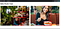A Simple TensorFlowJS Image Recognition Project | NodeJS & Coco SSD

An image recognition application built with TensorFlow.js.

TensorFlow.js is a popular library used to develop ML models for use in the JS runtimes such as the browser and Node.js. The true power of TensorFlow.js is its ability to train, and deploy the ML model right in the user’s device.

Project Walkthrough

The folder structure is quite simple. The files of interest are: ./index.html and ./src/index.js. The other javaScript file, i.e. ./images/index.js is only used to export the images (more detail on this later).

First we need to install all the dependencies listed below. The packages listed below except the first one should be standard for every TensorFlow.js project in Node.js. However, the first package i.e. @tensorflow-model/coco-ssd is only relevant for projects involving image recognition. TensorFlow.js has many such models for various projects. A complete list of the various models available can be found here. As mentioned above, our image recognition project uses the @tensorflow-model/coco-ssd model, more details on this model can be found here.

Once the dependencies are all installed, we should have a package.json file similar to the one below:

With the dependencies out of the way, we can write the html code. This project aims only to explain the basic concept, and this is represented by the simple html file (index.html).

The next step is the most important, we code up ./src/index.js. Here we load the Coco-ssd model, and we the detect the available landmarks on the supplied/candidate images.

First, we import all dependencies, and implement the parseImageImport function. The parseImageImport function receives the candidate images as a module, and returns an Image() object representing each supplied candidate image.

Second, we code up two additional functions. detectContour() is responsible for drawing the markings on the predictions, it expects an image object, a context (i.e. the result of the getContext API, see explanation here), and a predictions array (this is the result of the Coco-Ssd tensorFlow model, more on this later). drawImage() is responsible for two things. First, it draws the image on the canvas, and second, it has an eventListener that calls the detectContour function when the canvas is clicked.

Lastly, we have an IIFE that brings it all together. It loops through the result of the parseImageImport function (i.e. the candidate images). For every candidate image, it creates a new canvas and appends the canvas to the html body. It also runs loads the CocoSsd model and performs predictions for every candidate image. The predications are supplied as a parameter to the helper function mentioned above, drawImage.

I promised to show the contents of ./images/index.js. This file is merely a function exporting the candidate image files as a module. See below contents of ./images/index.js.

ResultApplication showing candidate images annotated with predictions. Candidate image source: Unsplash

In conclusion

This article explained how to build a simple image recognision web application using TensorFlow.js in Node.js environment. The Github repository can be found here.

Engineer